# Sliding Window Technique

### What is Sliding Window Technique?

Before discussing sliding window technique, let’s assume if you need to traverse a array one element at a time, how will you do it?

Something like this:

```if array = {1,2,3,4,5}
The traversal sequence will look like
the highlighted element at each step:

step 1: {1,2,3,4,5}
step 2: {1,2,3,4,5}
step 3: {1,2,3,4,5}
step 4: {1,2,3,4,5}
step 5: {1,2,3,4,5}```

But as the name suggest, in case of sliding window, instead of traversing the array 1 by 1, we would traverse a block of size K in the first step, and then simply shift the block ahead by one position at each step. You can visualise this as a sliding glass pane of a window frame. That’s why it is called sliding window.

For Example:

```if array = {1,2,3,4,5}, and K = 2
The traversal sequence of sliding a
window by size 2 would look like:

step 1: {1,2,3,4,5}
step 2: {1,2,3,4,5}
step 3: {1,2,3,4,5}
step 4: {1,2,3,4,5}```
##### What is the benefit of using sliding window technique?

Before we discuss the benefit, can you spot the difference between the above two examples?

The difference is in the number of steps. In the first example of traditional traversal, there are 5 steps whereas in the second one, we just have 4 steps.

Although it doesn’t make a lot of sense in the above example as the time complexity would be same, but in a lot of problems, sliding window techniques helps us to remove using nested loop by replacing it with a single loop, there by dramatically reducing the time complexity.

Let us look at one relevant example: We are given an array of n integers where we need to find the maximum sum of k consecutive elements.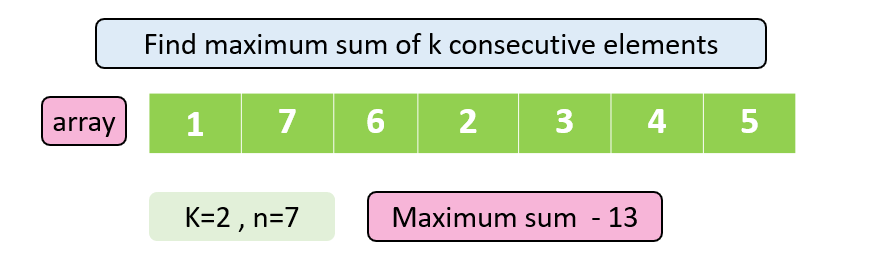Traditional Brute Force approach (Without Sliding Window Technique).

The brute force approach for the given problem is to go to every index of the array and calculate the sum by running the inner nested loop k times for that index and then find the maximum sum consecutively.

For the given array:

```Arr = {1,7,6,2,3,4,5}

k = 2```

Let us consider maxsum as INT_MIN.

The most basic approach that strikes in the first go is to use two nested loops. The outer loop is to traverse the array one by one, and the inner loop to calculate maximum of K consecutive elements at each step.

0th index:

• Run the loop there 2 ( k) times
• So the currsum will be 8 and the maxsum will be updated as 8.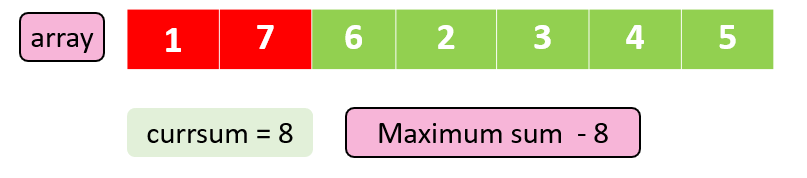1st Index:

• After running loop here for 2 times, we will get currsum as 13
• Since maxsum is smaller than currsum. So we will update the maxsum as 13.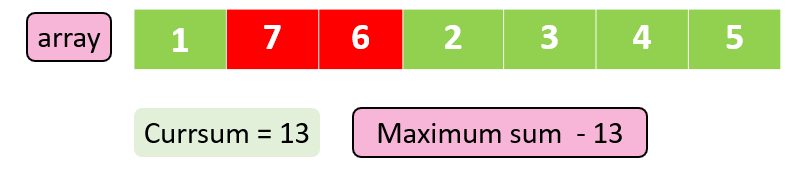2nd index:

• After running loop here for 2 times, we will get currsum as 8
• Since maxsum is already greater than currsum, we will not update it.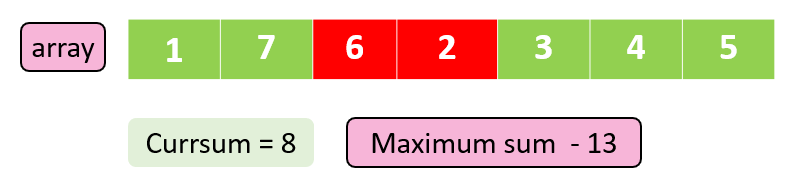Similarly, we go for other indices and find that maxsum – 13.

## C++ Code

``````#include <bits/stdc++.h>
using namespace std;
int findmaxconssum(int arr[], int n, int k)
{
int maxsum = INT_MIN;
for (int i = 0; i < n - k; i++)
{
int currsum = 0;
for (int j = 0; j < k; j++)
{
currsum = currsum + arr[i + j];
}
maxsum = max(maxsum, currsum);
}
return maxsum;
}
int main()
{
int arr[] = {1, 7, 6, 2, 3, 4, 5};
int n = 7;
int k = 2;
cout<<findmaxconssum(arr, n, k) << endl;
return 0;
}
``````

Output: 13

Time Complexity – O(n*k)

Space complexity – O(1)

## Java Code

``````import java.util.*;
public class Main {
public static int findmaxconssum(int arr[],int n,int k)
{
int maxsum=Integer.MIN_VALUE;
for(int i=0;i<n-k;i++)
{
int currsum=0;
for(int  j=0;j<k;j++)
currsum+=arr[j+i];
maxsum=Math.max(maxsum,currsum);
}
return maxsum;
}
public static void main(String args[]) {
int arr[]={1,7,6,2,3,4,5};
int n=7;
int k=2;
System.out.println(findmaxconssum(arr,n,k));
}
}
``````

Output: 13

Time Complexity – O(n*k)

Space complexity – O(1)

### Solution 2: (Sliding Window Technique)

Can you think how you can remove the second loop or the inner loop in the above brute force approach by using the sliding window technique?

Observations:

• The elements are consecutive in every window.
• The next window overlaps almost entirely with previous window except the first element of previous window.
• While moving to next window, we are adding just one new element, removing one old element from the sum of previous window.

Visually it will appear something like: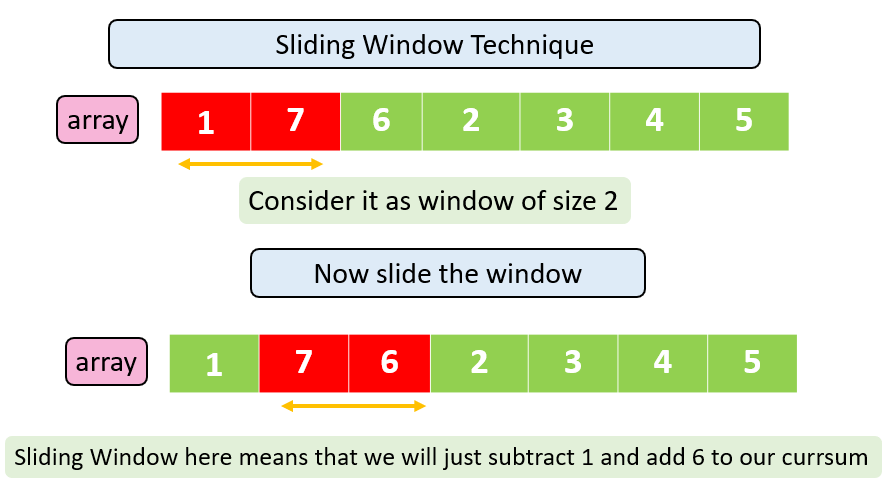```For the given array , Arr = {1,7,6,2,3,4,5}, and K = 2.

Let us assume currsum = 0

And maxsum = INT_MIN```

So for the first window of size K, our currsum will be 8 and maxsum will also be 8.

For the next window of size K, we can calculate currsum simply by:

• removing first element.
• adding the new element.

Hence, updated currsum for second window will be:

```currsum = currsum -  1 + 6
= 8 - 1 + 6
= 13

Therefore, maxsum will be updated to 13.```

Similarly, we will keep on sliding the window one by one, and calculate the max sum of all windows of size K.

## C++ Code

``````#include <bits/stdc++.h>
using namespace std;
// Using sliding window technique
int findmaxconssum(int arr[], int n, int k)
{
int currsum=0;
for(int i=0;i<k;i++)
{
currsum+=arr[i];
}
int maxsum=currsum;
for(int i=k;i<n-k;i++)
{
currsum-=arr[i-k];
currsum+=arr[i];
maxsum=max(maxsum,currsum);
}
return maxsum;
}
int main()
{
int arr[] = {1, 7, 6, 2, 3, 4, 5};
int n = 7;
int k = 2;
cout << findmaxconssum(arr, n, k) << endl;
return 0;
}
``````

Output: 13

Time complexity – O(n)

Space complexity – O(1)

## Java Code

``````import java.util.*;
public class Main {
// Using sliding window technique
public static int findmaxconssum(int arr[], int n, int k) {
int currsum = 0;
for (int i = 0; i < k; i++) {
currsum += arr[i];
}
int maxsum = currsum;
for (int i = k; i < n - k; i++) {
currsum -= arr[i - k];
currsum += arr[i];
maxsum = Math.max(maxsum, currsum);
}
return maxsum;
}
public static void main(String args[]) {
int arr[] = {1, 7, 6, 2, 3, 4, 5};
int n = 7;
int k = 2;
System.out.println(findmaxconssum(arr, n, k));
}
}
``````

Output: 13

Time complexity – O(N)

Space complexity – O(1)

Special thanks to Gurmeet Singh for contributing to this article on takeUforward. If you also wish to share your knowledge with the takeUforward fam, please check out this article